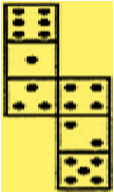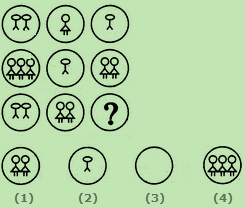# Indian Talent Olympiad - NMITO PDF Sample Papers for Class 8

Resources:

Class 8 sample paper & practice questions for National Mathematics Indian Talent Olympiad (NMITO) level 1 are given below. Syllabus for level 1 is also mentioned for these exams. You can refer these sample paper & quiz for preparing for the exam.Q.1 Q.2 Q.3 Q.4 Q.5 Q.6 Q.7 Q.8 Q.9 Q.10
 Q.1 How many dots lie opposite to the face having three dots, when the given figure is folded to form a cube?a) 2 b) 4 c) 6 d) 8
 Q.2 Factorise 2x²y² + 5xy - 12 a) 3xy - 12x + 9y b) (2xy - 3)(xy + 2) c) 4y - 3xy d) (2xy - 3)(xy + 4)
 Q.3 Y is directly proportional to x2, x = 2 and y = 20, value of x when y = 1.25 is _______________. a) 0.3 b) 0.6 c) 0.5 d) 0.8
 Q.4 If in the word 'DISTURBANCE', the first letter is interchanged with the last letter, the second letter is interchanged with the tenth letter and so on, which letter would come after the letter T in the newly formed word? a) N b) C c) S d) E
 Q.5 Both U and V vary inversely with each other. When U is 10, V is 6, which of the following is a possible pair of corresponding values of U and V? a) 12 and 15 b) 14 and 15 c) 25 and 27 d) 45 and 27
 Q.6 In equation 9x -12k = 0. For x = 4, value of k is: a) 6 b) 5 c) 3 d) 8
 Q.7 What will be the length of third side of a right angled triangle whose hypotenuse is 5cm and one of the side is 3 cm? a) 2 b) 3 c) 4 d) 5
 Q.8 A geometric representation showing the relationship between a whole and its parts is a: a) Pie Chart b) Histogram c) Bar Graph d) Pictograph
 Q.9 Select a suitable figure from the four alternatives that would complete the figure matrix.a) 1 b) 2 c) 3 d) 4
 Q.10 Two positive numbers differ by 5 and square of their sum is 169 are: a) 2,4 b) 5,6 c) 4,9 d) 3,7Sample PDF of Indian Talent Olympiad - National Mathematics Indian Talent Olympiad (NMITO) PDF Sample Papers for Class 8: